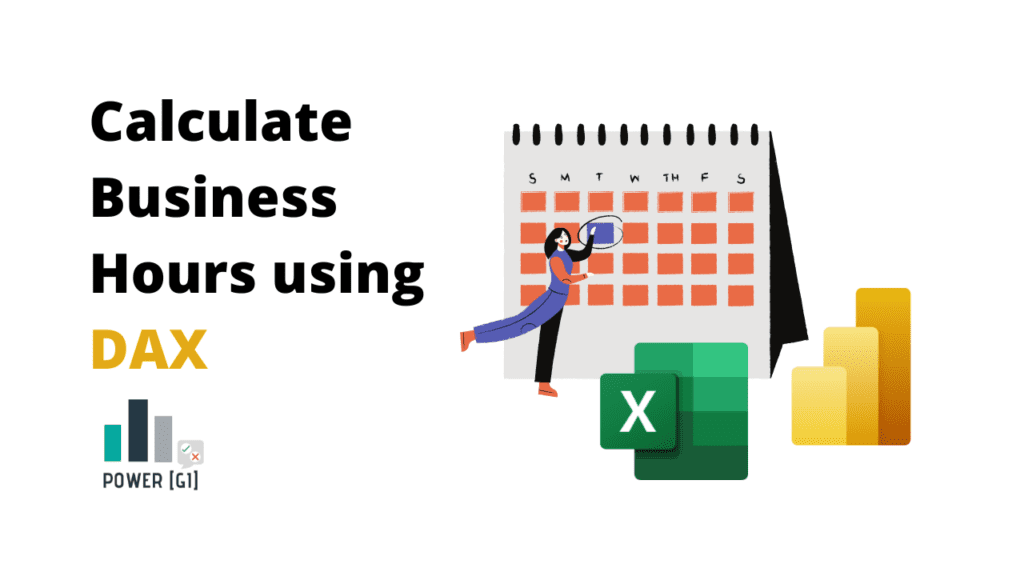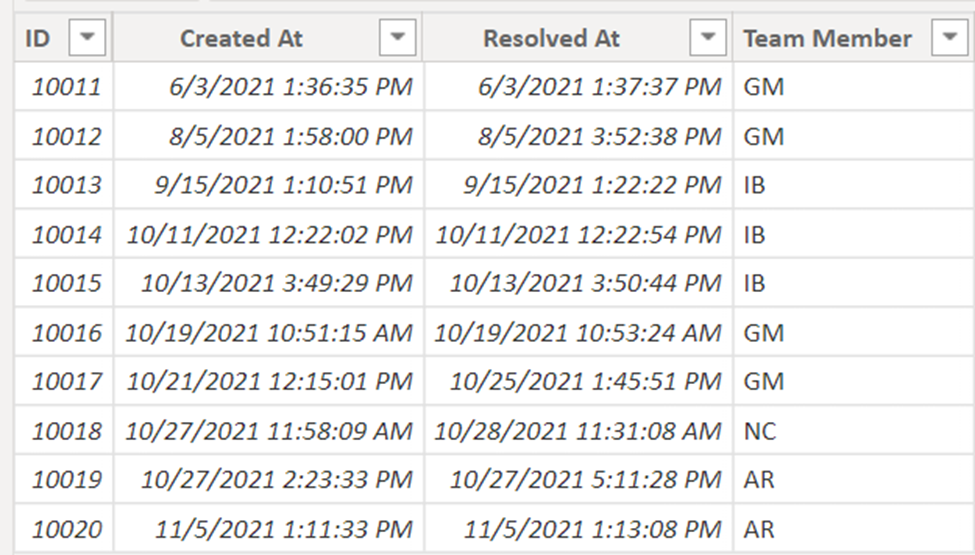# Calculate Business / Working Hours using DAX

We have the following table with Request ID, date time value on when it was created and when it was resolved, and we need to calculate the hours it takes for each request to be completed.

Our team works from 8 AM to 5 PM, Monday to Friday.First, make sure you create a calendar table for your data, for simplicity I created one from 1/1/2021 to 12/31/2022.

```				```
//if Saturday or Sunday, then workday = 0, else workday = 1
Workday = If(Weekday(Calendar_Table[Date]) = 7 || Weekday(Calendar_Table[Date]) = 1, 0,1)

//indicate start time of shift, we’ll add the date of each day to this hour
Start =
var workDayStart = time(8,0,0)
var eachdate = DATEVALUE(Calendar_Table[Date])
return eachdate + workDayStart

//indicate end time of shift, we’ll add the date of each day to this hour
End =
var workEndStart = time(17,0,0)
var eachdate = DATEVALUE(Calendar_Table[Date])
return eachdate + workEndStart

```
```

This is how your calendar table should look